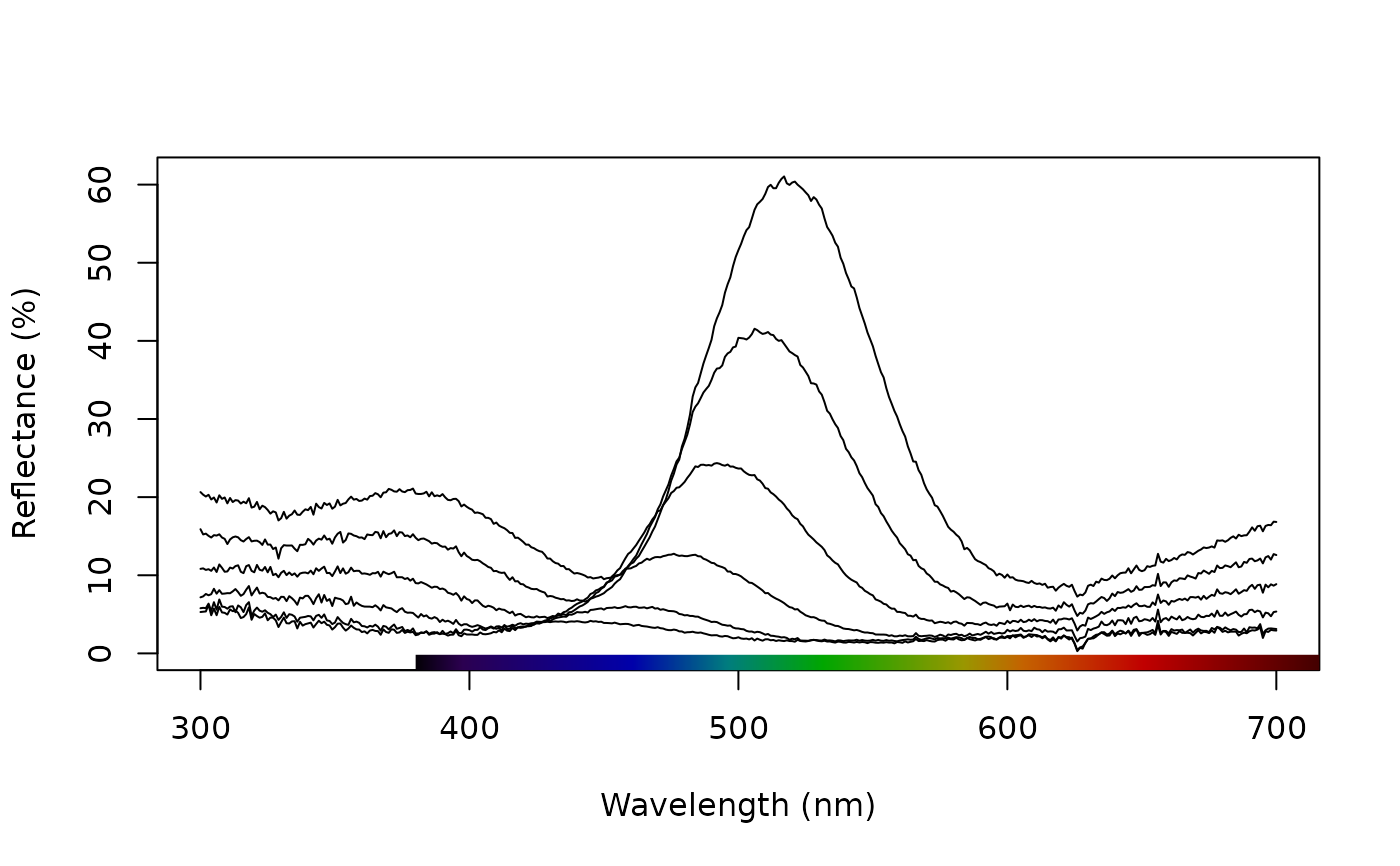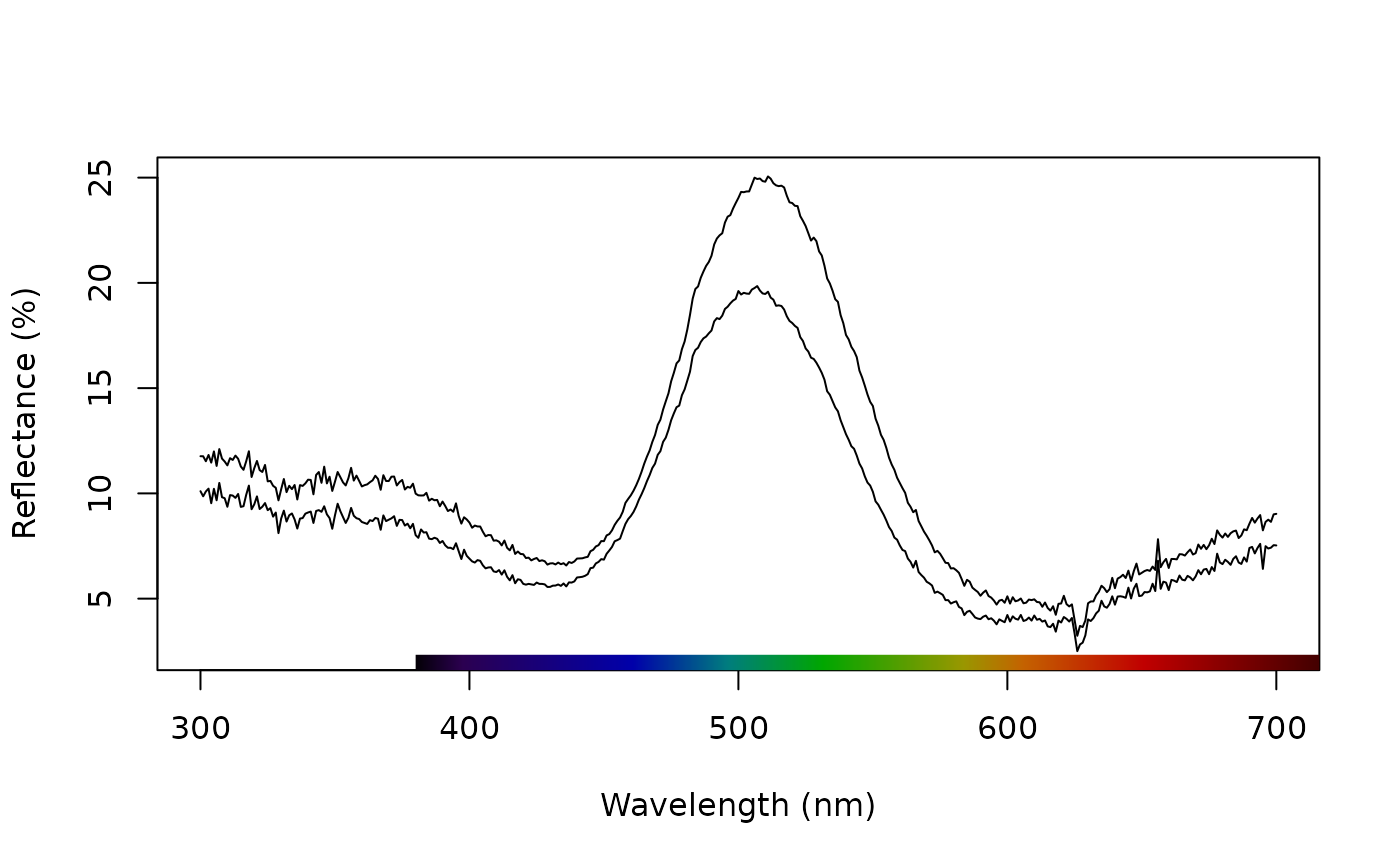Combines spectra (by taking the average, for example) according to an index or a vector of identities.

aggspec(rspecdata, by = NULL, FUN = mean, trim = TRUE)

## Arguments

rspecdata

(required) a data frame, possibly of class rspec, which contains a column containing a wavelength range, named 'wl', and spectra data in remaining columns.

by

(required) either a single value specifying the range of spectra within the data frame to be combined (for example, by = 3 indicates the function will be applied to groups of 3 consecutive columns in the spectra data frame); a vector containing identifications for the columns in the spectra data frame (in which case the function will be applied to each group of spectra sharing the same identification); or a list of vectors, e.g., by = list(sex, species).

FUN

the function to be applied to the groups of spectra. (defaults to mean())

trim

logical. if TRUE (default), the function will try to identify and remove numbers at the end of the names of the columns in the new rspec object.

## Value

A data frame of class rspec containing the spectra after applying the aggregating function.

## Examples

data(teal)

# Average every two spectra
teal.sset1 <- aggspec(teal, by = 2)
plot(teal.sset1)# Create factor and average spectra by levels 'a' and 'b'
ind <- rep(c("a", "b"), times = 6)
teal.sset2 <- aggspec(teal, by = ind)

plot(teal.sset2)